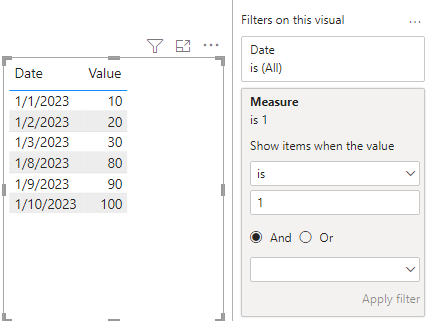cancel
Showing results for
Did you mean:Frequent Visitor

## 10 ten and bottom 10

Hello,

I am trying to find a Dax formula to show the 10 top and the 10 lowest entries. This chart that I am using has 7 collumns and each collumns has to have its top and lowest numbersI am trying todo this with out filters, and bookmarks. Would love some help.

Thanks

1 ACCEPTED SOLUTIONCommunity Support

I made an example where the top 3 and bottom 3 appear at the same time.

Sample data:``````Measure =
Var _table1=SUMMARIZE(TOPN(3,ALL('Table'),[Value],DESC),[Value])
Var _table2=SUMMARIZE(TOPN(3,ALL('Table'),[Value],ASC),[Value])
Var _union=UNION(_table1,_table2)
Return
IF(MAX('Table'[Value]) in _union,1,0)``````Result:Is this the result you expect?

Best Regards,

Community Support Team _Charlotte

If this post helps, then please consider Accept it as the solution to help the other members find it more quickly.Community Support

I made an example where the top 3 and bottom 3 appear at the same time.

Sample data:``````Measure =
Var _table1=SUMMARIZE(TOPN(3,ALL('Table'),[Value],DESC),[Value])
Var _table2=SUMMARIZE(TOPN(3,ALL('Table'),[Value],ASC),[Value])
Var _union=UNION(_table1,_table2)
Return
IF(MAX('Table'[Value]) in _union,1,0)``````Result:Is this the result you expect?

Best Regards,

Community Support Team _Charlotte

If this post helps, then please consider Accept it as the solution to help the other members find it more quickly.